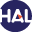Home page > 18. Volume 12 (2015) > Convergence of a finite volume scheme for a corrosion model

# Convergence of a finite volume scheme for a corrosion model

Tuesday 9 June 2015,

Abstract

In this paper, we study the numerical approximation of a system of partial differential equations describing the corrosion of an iron based alloy in a nuclear waste repository. In particular, we are interested in the convergence of a numerical scheme consisting in an implicit Euler scheme in time and a Scharfetter-Gummel finite volume scheme in space.

Keywords : finite volume scheme, corrosion model, convergence analysis, drift-diffusion system

Paper presented by: Pascal OmnesVoir sur HAL : https://hal.archives-ouvertes.fr/hal-01082041v3